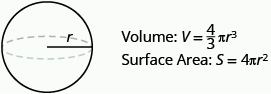Finding the Volume and Surface Area of a Sphere

Learning Outcomes

• Find the volume and surface area of a sphere

A sphere is the shape of a basketball, like a three-dimensional circle. Just like a circle, the size of a sphere is determined by its radius, which is the distance from the center of the sphere to any point on its surface. The formulas for the volume and surface area of a sphere are given below.

Showing where these formulas come from, like we did for a rectangular solid, is beyond the scope of this course. We will approximate $\pi$ with $3.14$.

Volume and Surface Area of a Sphere

For a sphere with radius $r\text{:}$example

A sphere has a radius $6$ inches. Find its 1. volume and 2. surface area.

Solution
Step 1 is the same for both 1. and 2., so we will show it just once.

 Step 1. Read the problem. Draw the figure and label it with the given information.1. Step 2. Identify what you are looking for. the volume of the sphere Step 3. Name. Choose a variable to represent it. let $V$ = volume Step 4. Translate. Write the appropriate formula. $V=\Large\frac{4}{3}\normalsize\pi {r}^{3}$ Step 5. Solve. $V\approx \Large\frac{4}{3}\normalsize\left(3.14\right){6}^{3}$ $V\approx 904.32\text{cubic inches}$ Step 6. Check: Double-check your math on a calculator. Step 7. Answer the question. The volume is approximately $904.32$ cubic inches.
 2. Step 2. Identify what you are looking for. the surface area of the cube Step 3. Name. Choose a variable to represent it. let S = surface area Step 4. Translate. Write the appropriate formula. $S=4\pi {r}^{2}$ Step 5. Solve. $S\approx 4\left(3.14\right){6}^{2}$ $S\approx 452.16$ Step 6. Check: Double-check your math on a calculator Step 7. Answer the question. The surface area is approximately $452.16$ square inches.

example

A globe of Earth is in the shape of a sphere with radius $14$ centimeters. Find its 1. volume and 2. surface area. Round the answer to the nearest hundredth.

try it

In the next video we show an example of how to find the surface area of a sphere.

And in our final video we show an example of how to find the volume of a sphere given it’s diameter.